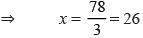Courses

# Olympiad Test: Linear Equations In One Variable -1

## 15 Questions MCQ Test Mathematics Olympiad Class 7 | Olympiad Test: Linear Equations In One Variable -1

Description
This mock test of Olympiad Test: Linear Equations In One Variable -1 for Class 7 helps you for every Class 7 entrance exam. This contains 15 Multiple Choice Questions for Class 7 Olympiad Test: Linear Equations In One Variable -1 (mcq) to study with solutions a complete question bank. The solved questions answers in this Olympiad Test: Linear Equations In One Variable -1 quiz give you a good mix of easy questions and tough questions. Class 7 students definitely take this Olympiad Test: Linear Equations In One Variable -1 exercise for a better result in the exam. You can find other Olympiad Test: Linear Equations In One Variable -1 extra questions, long questions & short questions for Class 7 on EduRev as well by searching above.
QUESTION: 1

### Mr. Sharma left one-third of his property to his daughter, one- fourth to his son and the remainder to his wife. If his wife’s share is Rs 18000 what was the worth of Mr. Sharma’s total property?

Solution:

Let the total property be Rs x.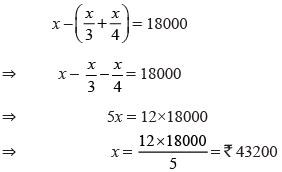QUESTION: 2

### In an examination, a student requires 40% of the total marks to pass. If Manoj gets 185 marks and fails by 15 marks what is the total marks?

Solution: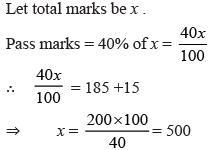QUESTION: 3

### 50 kg of an alloy of nickel and iron contains 60% nickel. How much nickel must be melted into it to make the alloy contain 75% of nickel?

Solution:

Quantity of nickel in 50 kg of alloy
= 60% of 50 kg = 30 kg
Let the required quantity of lead = x kg
Weight of new alloy = (30 + x ) kg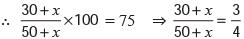⇒ 120 + 4x = 150 + 3x
⇒ 4x – 3x = 150 – 120 ⇒
x = 30

QUESTION: 4

The sum of digits of a two digit number is 9. If 27 is added to the number its digits get interchanged. What is the number?

Solution:

Let unit’s digit be x.
Ten’s digit = (9 – x)
Number = 10(9 – x) + 1× x = 90 –10x + x
∴ 90 – 9x + 27 = x × 10 + (9 – x)1.
⇒ 117 – 9x = 10 x + 9 –x
⇒ 117 – 9x = 9x + 9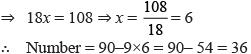QUESTION: 5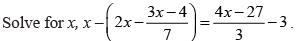Solution: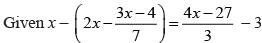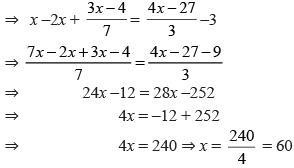QUESTION: 6

A bookseller earned a profit of 5% by selling a book for Rs 714. What is the cost price of the book?

Solution:

Let the cost price be Rs x.
∴ x + 5% of x = 714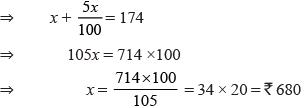QUESTION: 7

The length of a rectangle is three times of its breadth. Its perimeter is 128m, what is its length?

Solution:

Let the breadth of rectangle = x
Length = 3x
Perimeter of rectangle = 128
∴ 2(x + 3x) = 128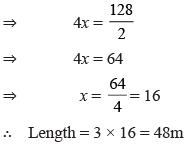QUESTION: 8

Ramesh travelled 3/5 of his journey by rail, 1/4 by a car, 1/8 by a bus and the remaining 4 km on foot. What is the length of total journey?

Solution:

Let the total journey be x km.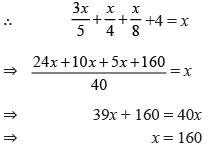QUESTION: 9

A number consists of two–digits whose sum is 8. If 18 is added to the number its digits are reversed. What is that number?

Solution:

Let the unit’s digit be x.
10’s digit = 8–x.
Number = 10(8–x) + 1×x
= 80 – 10x + x = 80 – 9x
and 80 –9x + 18 = 10 × x + (8–x) 1
⇒ 98 –9x = 10x + 8 –x
⇒ 98 –9x = 9x + 8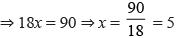Number = 80 – 9×5 = 80 – 45 = 35

QUESTION: 10

A number is as much greater than 21 as it is less than 71. What is that number?

Solution:

Let the number be x
∴ x – 21 = 71 – x
⇒ x + x = 71 + 21
⇒ 2x = 92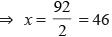QUESTION: 11

Two supplementary angles differ by 20°.  What is the measure of smaller angle?

Solution:

Let the smaller angle be x.
∴ Other angle = x + 20°
Now x  + x + 20° = 180°
⇒ 2x = 160°
⇒ x = 80°

QUESTION: 12

A number when multiplied by 5 is increased by 80. What is that number?

Solution:

Let the number be x.
∴ 5x = x +80
⇒ 5x – x = 80
⇒ 4x = 80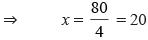QUESTION: 13

The sum of three consecutive odd numbers is 99. What is the difference of smallest and largest odd number?

Solution:

Let the three consecutive odd numbers be x, x +2, x + 4.
∴ x + x + 2 + x + 4 = 99
⇒ 3x + 6 = 99
⇒ 3x = 93
⇒ x  = 31
∴ The numbers are 31,33,35
Hence, difference = 35 – 31 = 4

QUESTION: 14

The ages of Simran and Ranjna are in the ratio 5:3. After 6 years their ages will be in the ratio 7:5. What is present age of Ranjna?

Solution:

Let Simran’s age = 5x
Ranjna’s age = 3x
After 6 years, Simran’s age = 5x + 6
Ranjna’s age = 3x + 6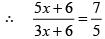⇒ 25x = 30 = 21x + 42
⇒ 25x –21x = 42 –30
⇒ 4x = 12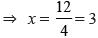∴ Present age of Ranjana = 3x
= 3 × 3 = 9 years

QUESTION: 15

Thrice a number when increased by 6 gives 84. What is that number?

Solution:

Let the number be x.
∴ 3x + 6 = 84
⇒ 3x = 84 –6
⇒ 3x = 78